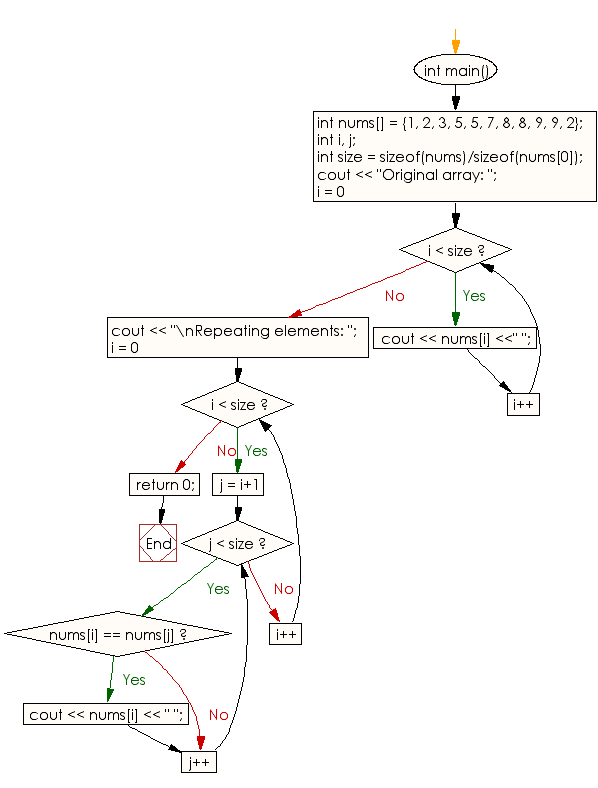﻿ C++ Exercises: Find the two repeating elements in a given array of integers - w3resourceC++ Exercises: Find the two repeating elements in a given array of integers

C++ Array: Exercise-21 with Solution

Write a C++ program to find the two repeating elements in a given array of integers.

Sample Solution:

C++ Code :

#include <iostream>
using namespace std;

int main()
{
int nums[] = {1, 2, 3, 5, 5, 7, 8, 8, 9, 9, 2};
int i, j;
int size = sizeof(nums)/sizeof(nums);
cout << "Original array: ";
for (i = 0; i < size; i++)
cout << nums[i] <<" ";
cout  << "\nRepeating elements: ";
for(i = 0; i < size; i++)
for(j = i+1; j < size; j++)
if(nums[i] == nums[j])
cout <<  nums[i] << " ";

return 0;

}

Sample Output:

Original array: 1 2 3 5 5 7 8 8 9 9 2
Repeating elements: 2 5 8 9

Flowchart:C++ Code Editor: